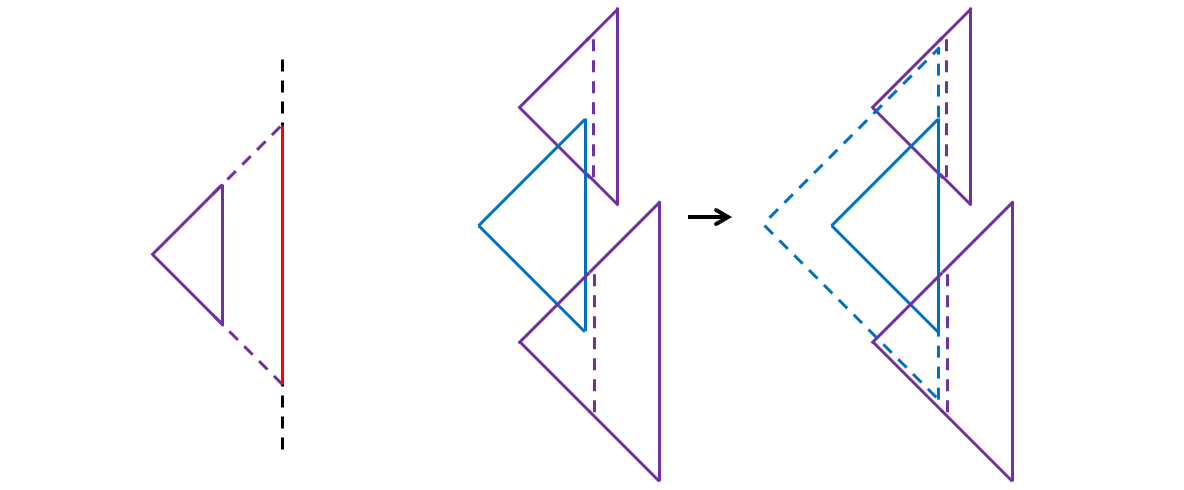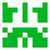# 2021.10月 月赛题解

changtiaoraplanqiu edited 1 年，3 月前

##### 花絮
• 先庆祝一下月赛暂时从双月赛变回月赛
• 第一次命题月赛, 所以十分害怕被大规模 ak, 就把题目都加强了一遍
• 包括把 D 题求 Bob 获胜的概率改成求 Alice 获胜的概率…没想到真能坑到人 @MAOoo
• F 题 感觉并不是很难, 验题的时候也确实是大家随手做的题, 不知道是不是赛前改小了 $n$ 的范围的缘故
• E 题原本是求值的和而不是异或… 感觉太简单了. 不过其实异或也挺无聊的…
• C 题是个比较基础的多项式EXP, 加进入增加一下数学浓度
• B 是加进去的签到
• A 题是个啥我也不知道
• 有可疑行为的选手已被打星, 如果觉得是被冤枉的可以来申诉

### 正文

#### A. Gold• 给定 $2m$ 个斜边竖直的等腰直角三角形（但是有左右两种朝向），求它们的面积并。

• 求 $2m$ 个斜着的正方形的面积并。

#### C. 洋葱圈

$$g_i(x) = (a_i - 1)!\frac{x^{a_i}}{a_i!} + (2a_i - 1)!\frac{x^{2a_i}}{2a_i!} + (3a_i - 1)!\frac{x^{3a_i}}{3a_i!} + … \ = \frac{x^{a_i}}{a_i} + \frac{x^{2a_i}}{2a_i} + \frac{x^{3a_i}}{3a_i} + …$$

$$g_i(x) + \frac{g_i(x)^2}{2!} + \frac{g_i(x)^3}{3!} + \dots = e^{g_i(x)}$$

$$e^{g_1(x)} \cdot e^{g_2(x)} \cdots e^{g_m(x)} \ = e^{g_1(x) + g_2(x) + \dots + g_m(x)}$$

#### D. 赌怪

$$\frac{\binom{2n}{n} \frac{1}{n + 1}}{\binom{2n}{n}} = \frac{1}{n + 1}$$

#### F. oh my cuber

• 第一版 $\frac{3n}{2} + 100$

• 第二版询问次数改到 $\frac{6n}{5}$, $n \ge 2e5$.

• 最后发现 $n$ 开到 $10000$ 都行.

int base = 1000;
int n = 13000;
for (int i = 1; i <= base / 2; i++) {
int cnt1 = ((double)i / base) * n;

long double p = 1;
int cnt2 = 0;
while (p > 0.0005) {
cnt2++;
p *= (1 - (1.0 * i / base) * (1.0 * i / base) * 0.5);
}
double x = 1.0 * (cnt1 + cnt2) / n;
dbg(i, cnt1, cnt2, x);
}F题我觉得第一步找到一个大于 $n/2$ 的数就行，期望是4步。记已知的最大数字是 $a$，这样每个数字在知道了模 $a$ 的余数之后只有2种可能，且如果小的那个已知那它必然是大的那个。这样还可以把 $a$ 更新的更大。而且每一次更新后 $n-a$ 的期望大小会除以2。感觉上最后的总期望步数在 $n + 2 \log n \sim n + 3 \log n$ 之间。我本地跑 $n = 20000$ 的最大步数不超过 $20035$。